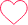# A cow and horse are bought for Rs.2,00,000. The cow is sold at a profit of 20% and the horse is sold a t a loss of 10%. The overall gain is Rs.4000, the Cost price of cow?

A cow and horse are bought for Rs.2,00,000. The cow is sold at a profit of 20% and the horse is sold a t a loss of 10%. The overall gain is Rs.4000, the Cost price of cow?

a) 130000            b) 80000             c) 70000               d) 120000

Overall profit = 4000200000×100=2%4000200000×100=2%
By applying alligation rule, we getSo cost price of the cow = 2/5 x 200000 = 80,000

### One comment on “A cow and horse are bought for Rs.2,00,000. The cow is sold at a profit of 20% and the horse is sold a t a loss of 10%. The overall gain is Rs.4000, the Cost price of cow?”

•maharshi panchal

preparation for tcs0